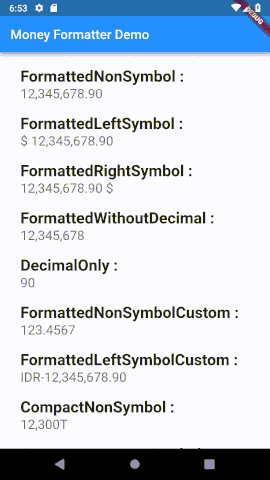## FlutterMoneyFormatter

FlutterMoneyFormatter is a Flutter extension to formatting various types of currencies according to the characteristics you like, without having to be tied to any localization.## Install

For complete steps in installing `FlutterMoneyFormatter` you can see in the Installation Guide.

## Usage

Import the library

``````import 'package:flutter_money_formatter/flutter_money_formatter.dart';
``````

## Getting Started

To be able to format your `double` value into the various formats you want, you first need to create a `FlutterMoneyFormatter` instance like the following:

``````FlutterMoneyFormatter fmf = FlutterMoneyFormatter(
amount: 12345678.9012345
);
``````

Note, the code above still uses the default configuration as explained here.

After that, you can request various results of the format as follows:

``````// normal form
print(fmf.output.nonSymbol); // 12,345,678.90
print(fmf.output.symbolOnLeft); // \$ 12,345,678.90
print(fmf.output.symbolOnRight); // 12,345,678.90 \$
print(fmf.output.fractionDigitsOnly); // 90
print(fmf.output.withoutFractionDigits); // 12,345,678

// compact form
print(fmf.output.compactNonSymbol) // 12.3M
print(fmf.output.compactSymbolOnLeft) // \$ 12.3M
print(fmf.output.compactSymbolOnRight) // 12.3M \$
``````

If you will use the output format several times, I strongly recommend that you initialize a variable as in the following example:

``````MoneyFormatterOutput fo = fmf.output;
``````

Or directly when initializing the `FlutterMoneyFormatter` instance as in the following example:

``````MoneyFormatterOutput fo = FlutterMoneyFormatter(
amount: 12345678.9012345
).output;
``````

So you can immediately take the value more easily as in the following example:

``````// normal form
print(fo.nonSymbol); // 12,345,678.90
print(fo.symbolOnLeft); // \$ 12,345,678.90
print(fo.symbolOnRight); // 12,345,678.90 \$
print(fo.fractionDigitsOnly); // 90
print(fo.withoutFractionDigits); // 12,345,678

// compact form
print(fo.compactNonSymbol) // 12.3M
print(fo.compactLeftSymbol) // \$ 12.3M
print(fo.compactRightSymbol) // 12.3M \$
``````

See demo section to get more info.

## Configurations

To adjust the format to suit your needs, you can set it through the `settings` parameter:

``````FlutterMoneyFormatter fmf = new FlutterMoneyFormatter(
amount: 12345678.9012345,
settings: MoneyFormatterSettings(
symbol: 'IDR',
thousandSeparator: '.',
decimalSeparator: ',',
symbolAndNumberSeparator: ' ',
fractionDigits: 3,
compactFormatType: CompactFormatType.sort
)
)
``````

Of course you are not required to initialize the entire configuration in the `settings`(`MoneyFormatterSettings`) parameter as in the example above. You can change one or more of the configurations above. This is because each configuration above is not mandatory and has a default value.

## `FlutterMoneyFormatter`

Property Names Data Type Descriptions
`amount` `double` Amount number that will be formatted.
`settings` `MoneyFormatterSettings` See here.
`output` `MoneyFormatterOutput` See here.
`comparator` `MoneyFormatterCompare` See here.
`copyWith` `FlutterMoneyFormatter` see here
`fastCalc` `FlutterMoneyFormatter` see here

## `MoneyFormatterSettings`

Configuration Property Data Type Default Value Description
`symbol` `String` `\$` (Dollar Sign) The symbol that will be used on formatted output.
`thousandSeparator` `String` `,` The character that will be used as thousand separator on formatted output.
`decimalSeparator` `String` `.` The character that will be used as decimal separator on formatted output.
`fractionDigits` `int` `2` The fraction digits that will be used on formatted output.
`symbolAndNumberSeparator` `String` `' '` (Space) The character that will be used as separator between formatted number and currency symbol.
`compactFormatType` `CompactFormatType` `CompactFormatType.short` See here.

## CompactFormatType

You can change the type of compact format like for million using `M` or `million`, or trillion using `T` or `trillion`. and so on. This type only supports two type as described below:

Value Description
`CompactFormatType.short` Used to make the compact format displayed using short text.
`CompactFormatType.long` Used to make the compact format displayed using long text.

## `MoneyFormatterOutput`

You can use formats that match your needs through properties found in the `MoneyFormatterOutput` instance.

Method Parameter Descriptions
`isLowerThan` `amount` Check current instance-amount is lower than [amount] or not.
`isGreaterThan` `amount` Check current instance-amount is greater than [amount] or not.
`isEqual` `amount` Check current instance amount is equal than [amount] or not.
`isEqualOrLowerThan` `amount` Check current instance amount is equal or lower than [amount] or not.
`isEqualOrGreaterThan` `amount` Check current instance amount is equal or greater than [amount] or not.

## `MoneyFormatterCompare`

Method Parameter Descriptions
`isLowerThan` `amount` Check current instance-amount is lower than [amount] or not.
`isGreaterThan` `amount` Check current instance-amount is greater than [amount] or not.
`isEqual` `amount` Check current instance amount is equal than [amount] or not.
`isEqualOrLowerThan` `amount` Check current instance amount is equal or lower than [amount] or not.
`isEqualOrGreaterThan` `amount` Check current instance amount is equal or greater than [amount] or not.

Example of using a comparator:

``````FlutterMoneyFormatter fmf = FlutterMoneyFormatter(amount: 12345678.9012345);
double comparerValue = 5678.9012;

print(fmf.comparator.isEqual(comparerValue)); // false
print(fmf.comparator.isGreaterThan(comparerValue)); // true

``````

## FastCalc

`fastCalc` is a function that can be used to perform various fast calculation processes that you might need. In implementing it, the `fastCalc` function gives the output of a`FlutterMoneyFormatter` instance so you can perform several calculation functions at once with the chaining method.

Function:

``````FlutterMoneyFormatter fastCalc({
@required FastCalcType type,
@required double amount
})
``````

Implementation:

``````FlutterMoneyFormatter fmf = FlutterMoneyFormatter(amount: 12345.678);
fmf.fastCalc(type: FastCalcType.addition, amount: 1.111);
fmf.fastCalc(type: FastCalcType.substraction, amount: 2.222);

print(fmf.output.nonSymbol); // 12,345.68
``````

Because it supports the chaining process, the example above can be shortened as follows:

``````FlutterMoneyFormatter fmf = FlutterMoneyFormatter(amount: 12345.678)
.fastCalc(type: FastCalcType.addition, amount: 1.111)
.fastCalc(type: FastCalcType.substraction, amount: 2.222);

print(fmf.output.nonSymbol);  // 12,345.68
``````

The `type` parameter used by the `fastCalc` function has the `FastCalcType` data type which is an enum. The following table is an explanation for the `FastCalcType` enum:

Index Name Description
0 addition Used to do addition calculations.
1 substraction Used to do substraction calculations.
2 multiplication Used to do multiplication calculations.
3 division Used to do division calculations.
4 percentageAddition Used to do the addition calculations base on percentage.
5 percentageSubstraction Used to do the substraction calculations base on percentage.

## Duplicating Instance

For some reasons, you may need to duplicate the `instance` and just need to change some configurations. To do that, you can use the `copyWith` method as below:

``````FlutterMoneyFormatter fmf = FlutterMoneyFormatter(amount: 12345678.9012345);

print(fmf.output.symbolOnLeft); // \$ 12,345,678.90
print(fmf.copyWith(symbol: 'IDR', symbolAndNumberSeparator: '-').output.symbolOnLeft); // IDR-12,345,678.90``````